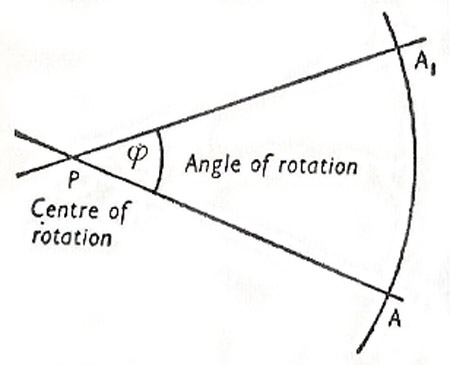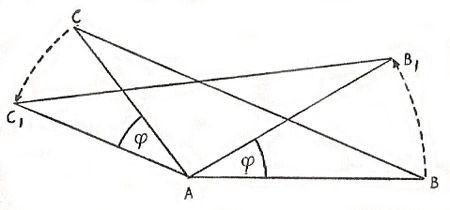A# rotationFig 1.Fig 2.

## Rotation in physics

In physics, rotation is the turning or spinning of a body, such as a planet, star, or galaxy, about an axis running through it.

### Rotation period

The rotation period of an object is the time taken for one complete turn (through 360 degrees) round the object's axis of rotation.

## Rotation in mathematics

In mathematics, rotation is a transformation in which a figure turns through a specific angle about a fixed point, called the center of rotation. The center of rotation may be inside or outside the figure that is being transformed. If the figure is turned anticlockwise, the rotation is considered positive, while a negative rotation turns the figure clockwise.

### Rotations in a plane

The rotations in a plane are determined by the point P in the plane which is unaltered by the rotation (center of rotation), and by the angle of rotation φ.

The image point A1 of a given point A can be constructed as follows. A circle with radius PA is described about P and the angle φ constructed on PA with vertex P. The intersection of the free arm of φ and the circle is the required image point A1 (Fig 1).

Example: Rotation of a triangle ABC about its vertex A through angle φ = 360°. The image of the triangle is AB1C1 (Fig 2).

## Rotations in space

A rotation in space is determined when an axis of rotation and an angle of rotation are given.